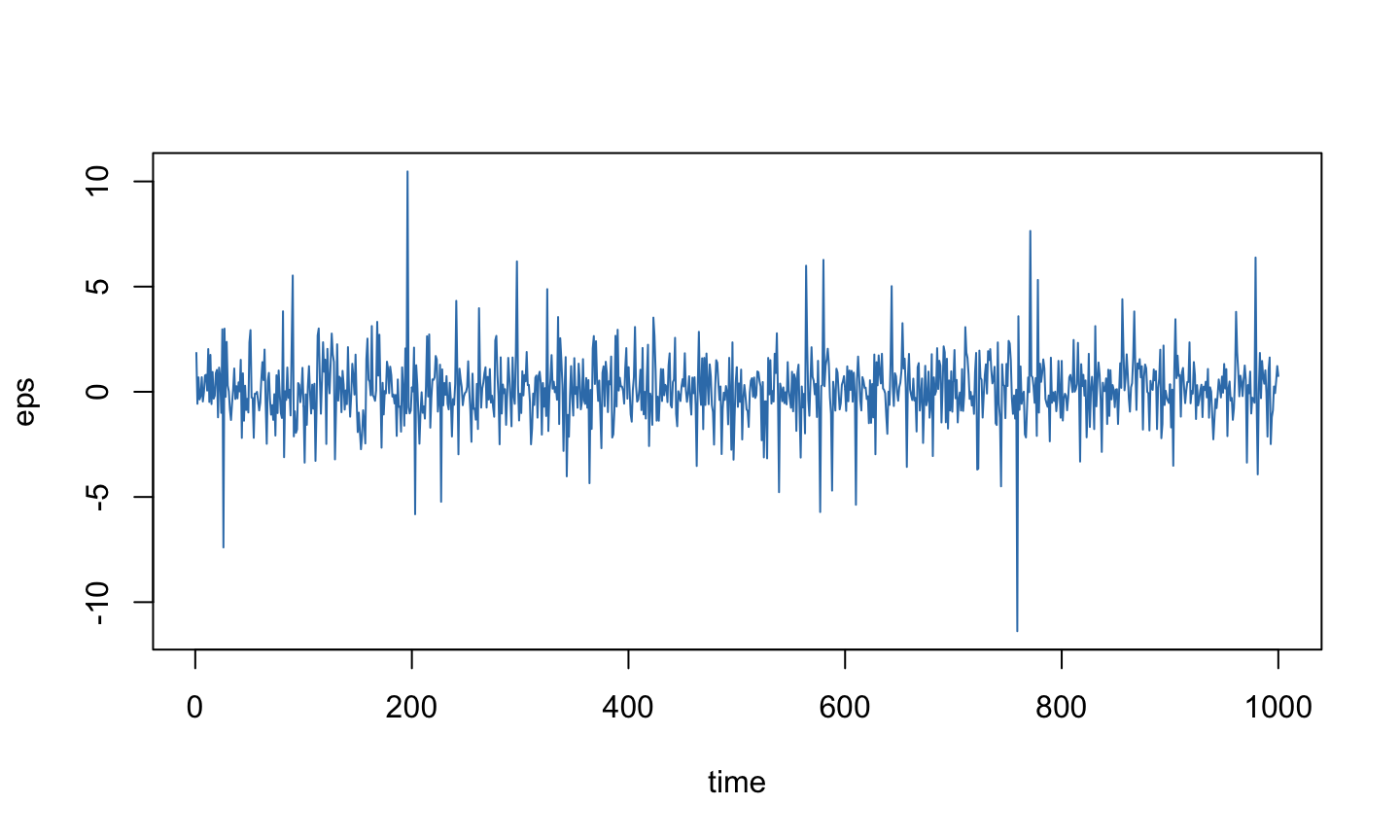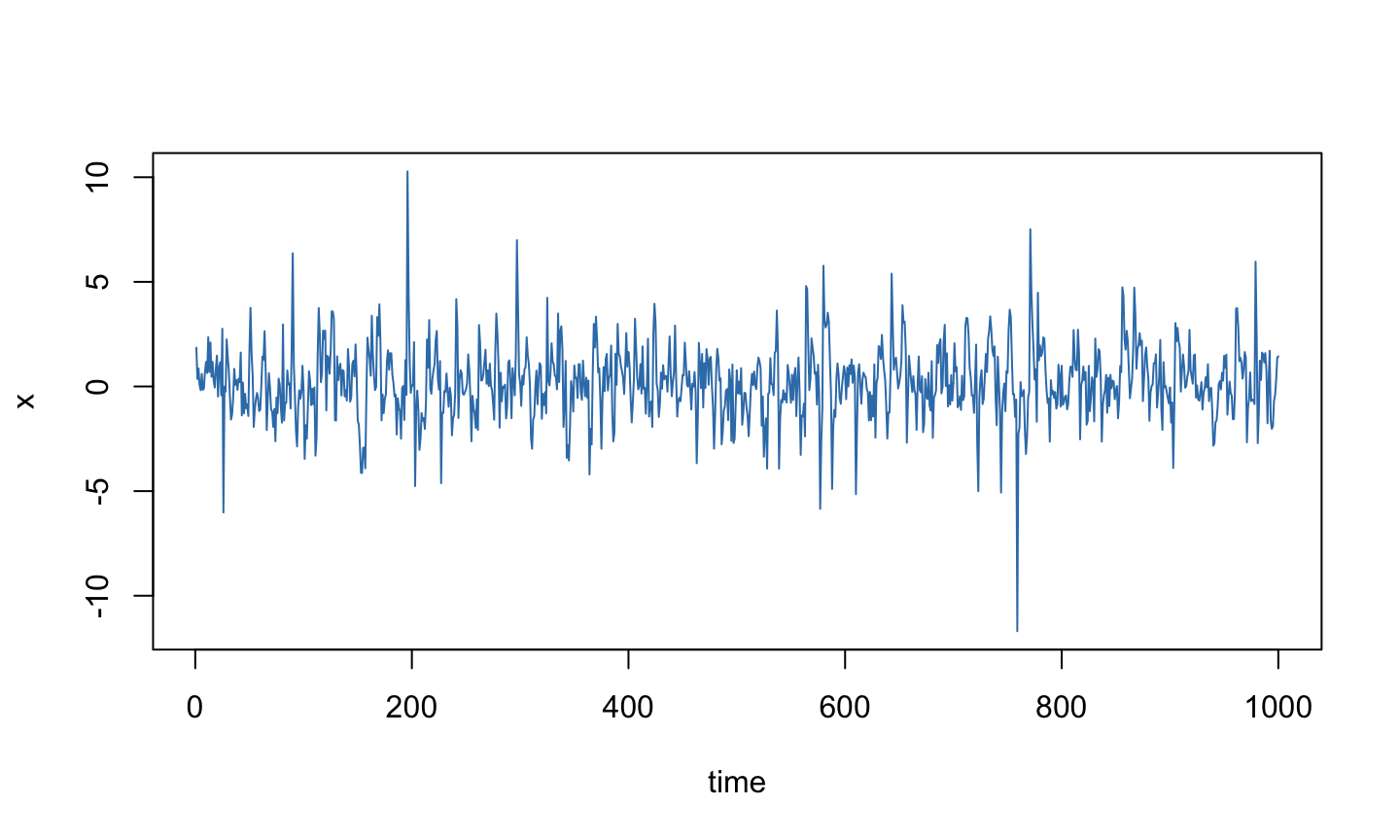February 28, 2017

## White Noise

```n <- 1000; time <- 1:n
eps <- rt(n, df=3) # t with 3 degrees of freedom (heavy-tailed)
plot(time, eps, type="l", col=2)```## AR(1), a = 0.5

```x <- filter(eps, 0.5, method="recursive")
plot(time, x, type="l", col=2)```## AR(1), a = -0.5

```x <- filter(eps, -0.5, method="recursive")
plot(time, x, type="l", col=2)```